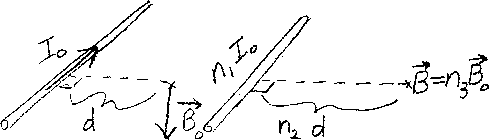Problem C12: Initially the current in a straight wire that is infinite in length is I0 (see left diagram above). The magnetic field vector a distance d from the wire is B0. Then the current is changed to n1I0 (see right diagram above). What is the magnetic field vector at a position that is n2d directly away from the wire? If the magnetic field vector is n3B0, what is n3?n1 = n2 = Input n3:
If you are currently in my class, you can record your grade by entering your name and student ID number (without the leading zeros) below and clicking on "record grade".
 First Name = Last Name = ID = Problem: